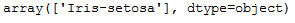# 1 Introduction

We have seen how to train and use different types of machine learning models. But how do we proceed when we have developed and trained a model with the desired performance? Due to the fact that the training of large machine learning models can sometimes take many hours, it is a good tip to save your trained models regularly so that you can access them later.

For this post the dataset Iris from the statistic platform “Kaggle” was used. You can download it from my “GitHub Repository”.

``````import pandas as pd

from sklearn.model_selection import train_test_split
from sklearn.svm import SVC

import seaborn as sns
import matplotlib.pyplot as plt

from sklearn.metrics import accuracy_score

#with joblib we'll safe our trained model
import joblib``````
``````iris = pd.read_csv("Iris_Data.csv")
iris = iris[['sepal_length', 'sepal_width', 'species']]

iris``````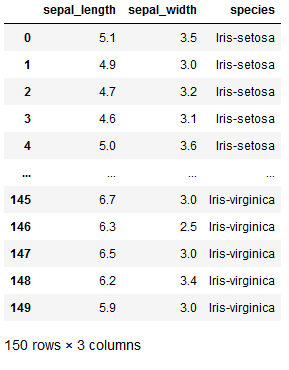# 3 Visualization of the data

``````g = sns.pairplot(iris, hue='species', markers='+')
plt.show()``````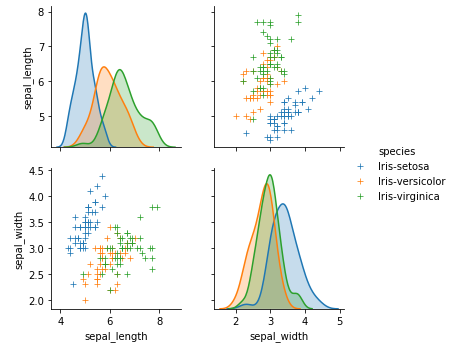# 4 Model training

``````x = iris.drop('species', axis=1)
y = iris['species']

trainX, testX, trainY, testY = train_test_split(x, y, test_size = 0.2)

clf = SVC(kernel='linear')
clf.fit(trainX, trainY)``````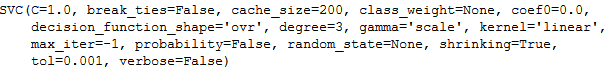``y_pred = clf.predict(testX)``
``````print('Accuracy: {:.2f}'.format(accuracy_score(testY, y_pred)))
print('Error rate: {:.2f}'.format(1 - accuracy_score(testY, y_pred)))``````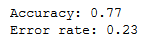# 5 Safe a model to disc

Now we have trained our model (here linear SVM). It’s time to safe the trained model to disc.

``````# save the model to disk

filename = 'final_svm_model.sav'
joblib.dump(clf, filename)``````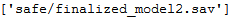Of course you can specify a different location under (here) filename.

# 6 Load a model from disc

The trained model can be reloaded at any later time.

``````# load the model from disk

filename = 'final_svm_model.sav'
``my_flower = [[4.0, 4.0]]``
``````my_pred = loaded_model.predict(my_flower)